# Description

The RandomIncreaseGen Generator can generate increasingly random numbers (whole and decimal) until the total sum of the generated numbers reaches the desired total (targetSum). The targetSum can be a positive or negative value.

Positive targetSum Example:

1,2, 5, 7 when targetSum = 15

Negative targetSum Example:

-8, -5, -4, -2, -1 when targetSum = -20

## When should RandomIncreaseGen be used?

• Any time a user wants to generate a random, finite set of numbers in increasing order that totals a specific value.

## When should RandomIncreaseGen not be used?

• Any time a user wants to generate a random set of numbers in no particular order where the total is irrelevant.

• Whenever a user wants to generate a random, finite set of numbers in decreasing order that totals a specific value.

## Generator Parameters

The following parameters may be configured for the RandomIncreaseGen Generator. Items with an asterisk* are required.

• targetSum* - Defines the target amount that all values must add up to based on the number of draws. The targetSum can be negative or positive.
• Positive Value -  The generated values will start at a lower number and increase in value. Example: 1, 4, 4, 5, 6 when targetSum = 15
• Negative Value - The generated values will start at the largest negative value and become closer to '0' as each value is generated. Example: -8, -5, -4, -2, -1 when targetSum = -20

• numberOfDraws* - Defines the finite number of values to be randomly generated in increasing order and must equal the sum defined in the targetSum parameter. The number of draws parameter value must be greater than '0'.
• format* - Defines the format of a value when it is decimal instead of an integer. Examples: #0.##, #0.00, #0
• seed - Defines an optional seed. Using a seed ensures the same random sequence is repeated each time test data is generated.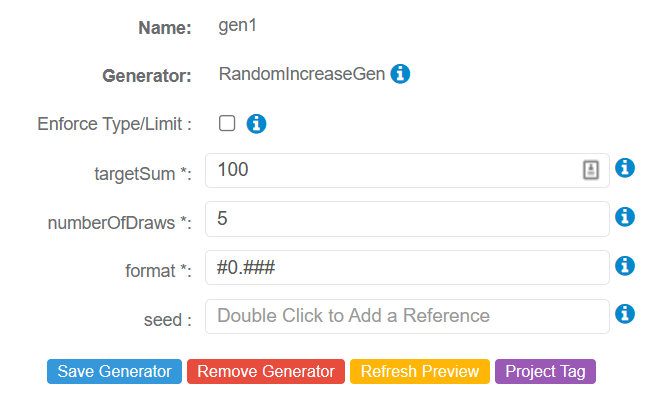## numberOfDraws Parameter and Domain loopCount

• The numberOfDraws parameter sets the Domain loopCount.

• If the Domain loopCount is greater than the numberOfDraws parameter value, record generation will stop at whatever value is entered as the number of draws within the RandomIncreaseGen Generator.

• Example - If the Domain loopCount is '20 "and the numberOfDraws parameter is '10', then only 10 records will be generated. The values generated for those 10 records will equal the targetSum parameter value.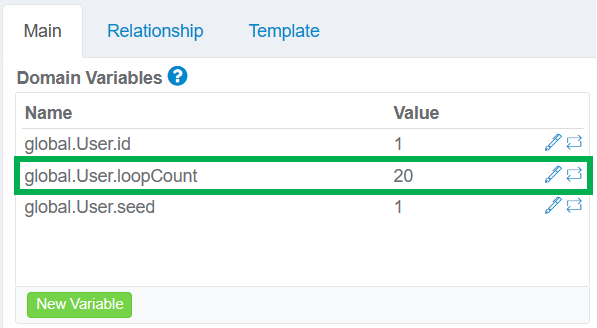numberOFDraws = 10The result is 10 records that total 100.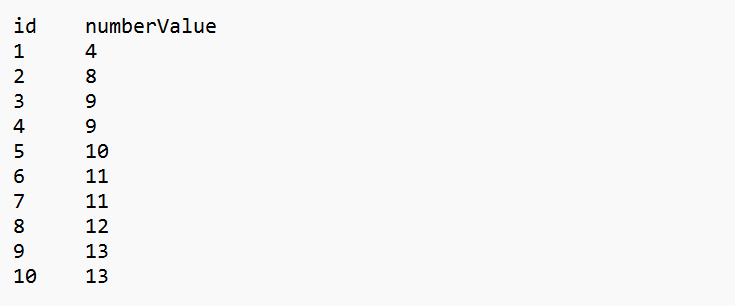## Example 1 - Generate 5 Random Values with a Target Sum of 50

For this example, the user wants to generate 5 random values, in increasing order, that total "50". The configuration would appear as shown below: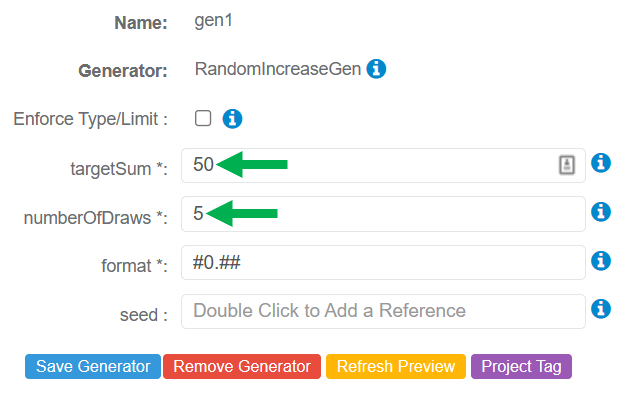Sample Output

Since 5 goes into 50 evenly in increasing order, the result will be whole numbers.## Example 2 - Generate 6 Random Values with a Target Sum of 100

For this example, the user wants to generate 6 random values, in increasing order, that total "100". The configuration would appear as shown below: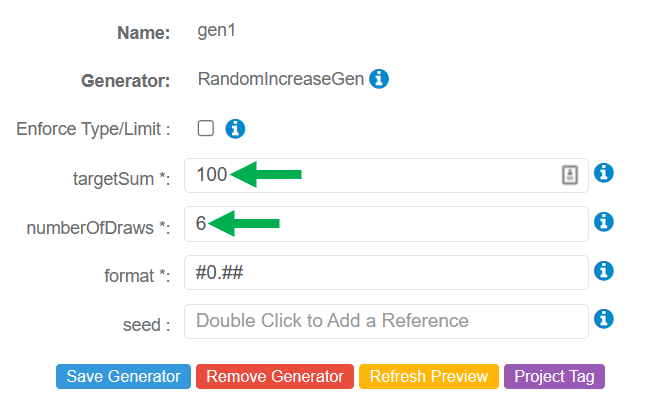Sample Output

In this example, decimal values will be generated since 6 does not evenly go into 100, in increasing order.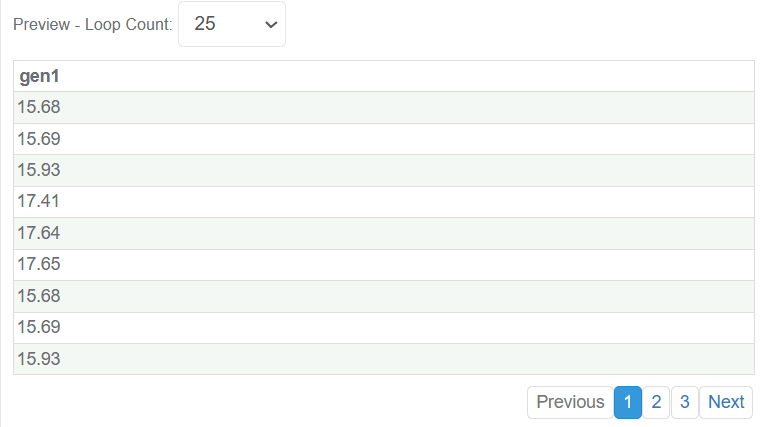## Example 3 - Generate 5 Random Values with a Target Sum of 30.25

For this example, the user wants to generate 5 random values, in increasing order, that total '30.25'. The configuration would appear as shown below: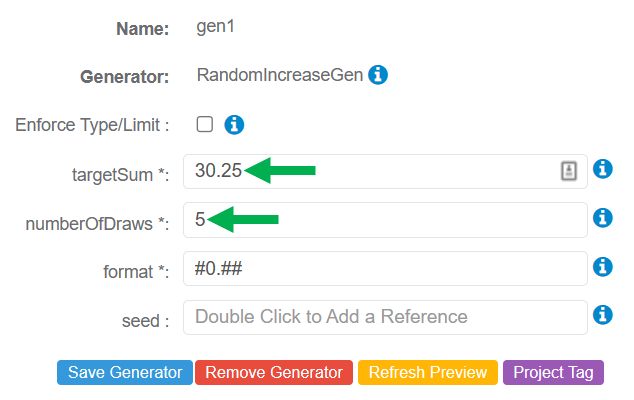Sample Output

The five generated numbers add up to 30.25 (as shown below):

• 5.23 + 5.92 = 11.15
• 11.15 + 6.05 = 17.20
• 17.20 + 6.18 = 23.38
• 23.38 + 6.87 = 30.25## Special Use Case - Using RandomIncreaseGen with RandomIncDecPeakGen

The numberOfDraws parameter within the RandomIncreaseGen and RandomIncDecPeakGen Generators must be the same value when assigned to one or more Attributes within the same Domain.

Example of Improper Configuration
In the example below, the Domain has two Attributes:

1. randomNumber - assigned RandomIncreasGen
2. randomNumber2 - assigned RandomIncDecPeakGen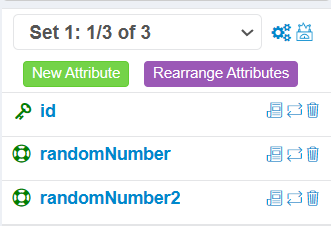The RandomIncreaseGen Generator has been assigned to the randomNumber Attribute. The numberOfDraws parameter has a value of '10".The RandomIncDecPeakGen Generator has been assigned to the randomNumber2 Attribute. The numberOfDraws parameter has a value of '5'. This Attribute Generator will be used to set the loopCount.Since the values are different for the numberOfDraws parameter, the following error will be received when generating test data with GenRocket Runtime.

To fix the error, the value must be the same for the numberOfDraws parameter in both Generators (RandomIncreaseGen and RandomIncDecPeakGen.